# Class 8 RD Sharma Solutions – Chapter 8 Division Of Algebraic Expressions – Exercise 8.4 | Set 2

• Last Updated : 30 Apr, 2021

### Question 19. Divide 30x4 + 11x3 – 82x2 – 12x + 48 by 3x2 + 2x – 4

Solution:

We have to divide 30x4 + 11x3 – 82x2 – 12x + 48 by 3x2 + 2x – 4

Hey! Looking for some great resources suitable for young ones? You've come to the right place. Check out our self-paced courses designed for students of grades I-XII

Start with topics like Python, HTML, ML, and learn to make some games and apps all with the help of our expertly designed content! So students worry no more, because GeeksforGeeks School is now here!

So by using long division method we get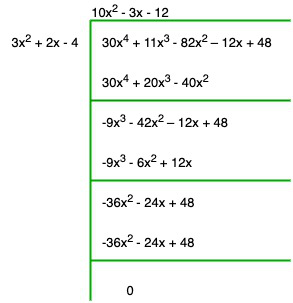Quotient = 10x2 – 3x – 12

Remainder = 0

### Question 20. Divide 9x4 – 4x2 + 4 by 3x2 – 4x + 2

Solution:

We have to divide 9x4 – 4x2 + 4 by 3x2 – 4x + 2

So by using long division method we get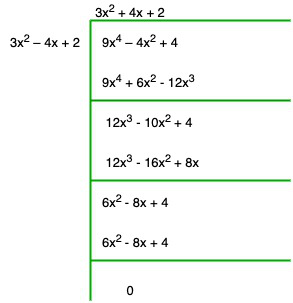Quotient = 3x2 + 4x + 2

Remainder = 0

### (i) Dividend = 14x2 + 13x – 15, Divisor = 7x – 4

Solution:

Dividing the Dividend by divisor, we get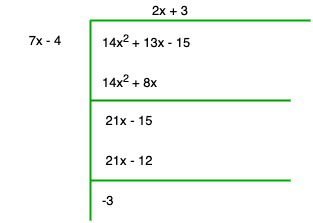Quotient = 2x + 3

Remainder = -3

### (ii) Dividend = 15z3 – 20z2 + 13z – 12, Divisor = 3z – 6

Solution:

Dividing the Dividend by divisor, we get

33z – 66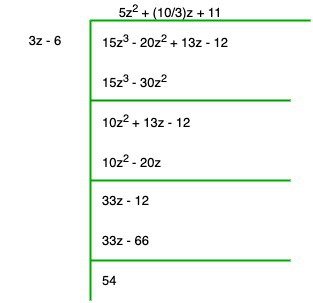Quotient = 5z2 + (10/3)z + 11

Remainder = 54

### (iii) Dividend = 6y5 – 28y3 + 3y2 + 30y – 9, Divisor = 2y2 – 6

Solution:

Dividing the Dividend by divisor, we get

3y2 – 9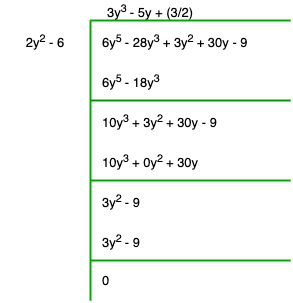Quotient = 3y3 – 5y + (3/2)

Remainder = 0

### (iv) Dividend = 34x – 22x3 – 12x4 – 10x2 – 75, Divisor = 3x + 7

Solution:

Dividing the Dividend by divisor, we get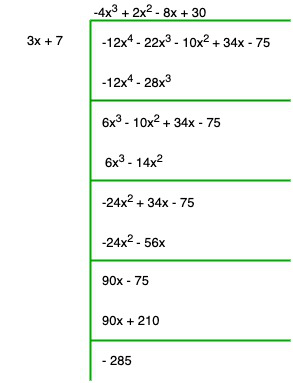Quotient = -4x3 + 2x2 – 8x + 30

Remainder = -285

### (v) Dividend = 15y4 – 16y3 + 9y2 – (10/3)y + 6, Divisor = 3y – 2

Solution:

Dividing the Dividend by divisor, we get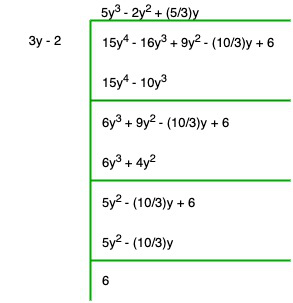Quotient = 5y3 – 2y2 + (5/3)y

Remainder = 6

### (vi) Dividend = 4y3 + 8y + 8y2 + 7, Divisor = 2y2 – y + 1

Solution:

Dividing the Dividend by divisor, we get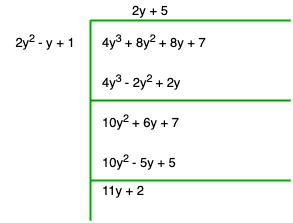Quotient = 2y + 5

Remainder = 11y + 2

### (vii) Dividend = 6y5 + 4y4 + 4y3 + 7y2 + 27y + 6, Divisor = 2y3 + 1

Solution:

Dividing the Dividend by divisor, we get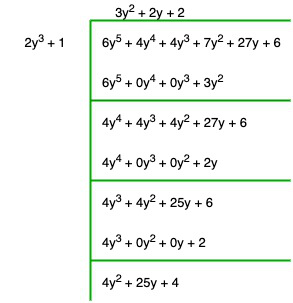Quotient = 3y2 + 2y + 2

Divisor = 4y2 + 25y + 4

### Question 22. Divide 15y4 + 16y3 + (10/3)y – 9y2 – 6 by 3y – 2 . Write down the coefficients of the terms in the quotient.

Solution:

We have to divide 15y4 + 16y3 + (10/3) y – 9y2 – 6 by 3y – 2

So by using long division method we get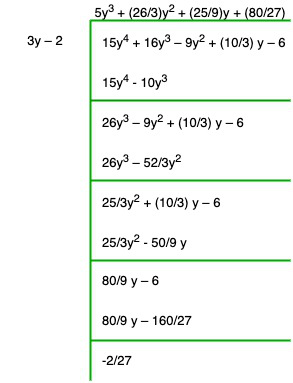Quotient = 5y3 + (26/3)y2 + (25/9)y + (80/27)

Remainder = (-2/27)

Co-efficient of y3 is 5

Co-efficient of y2 is 26/9

Co-efficient of y is 25/9 and,

Constant term = 80/27

### (i) x + 6 is a factor of x2 – x – 42

Solution:

Dividing x2 – x – 42 by x + 6, we get

-7x – 42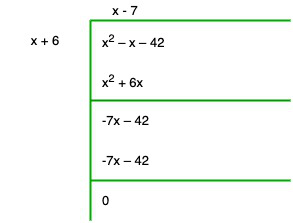Remainder = 0

Therefore, x + 6 is a factor of x2 – x – 42

### (ii) 4x – 1 is a factor of 4x2 – 13x – 12

Solution:

On dividing 4x2 – 13x – 12 by 4x – 1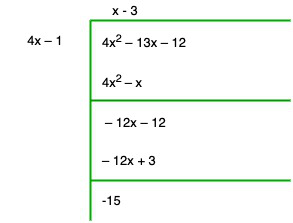Remainder = -15

Therefore, 4x-1 is not a factor of 4x2 – 13x – 12

### (iii) 2y – 5 is a factor of 4y4 – 10y3 – 10y2 + 30y – 15

Solution:

On dividing 4y4 – 10y3 – 10y2 + 30y – 15 by 2y – 5, we get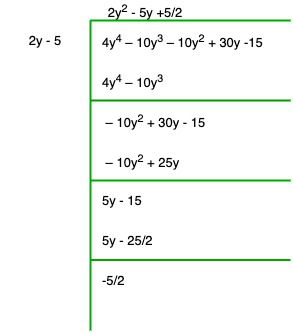Remainder = -5/2

Therefore, 2y – 5 is not a factor of 4y4 – 10y3 – 10y2 + 30y – 15

### (iv) 3y2 + 5 is a factor of 6y5 + 15y4 + 16y3 + 4y2 + 10y – 35

Solution:

On dividing 6y5 + 15y4 + 16y3 + 4y2 + 10y – 35 by 3y2 + 5, we get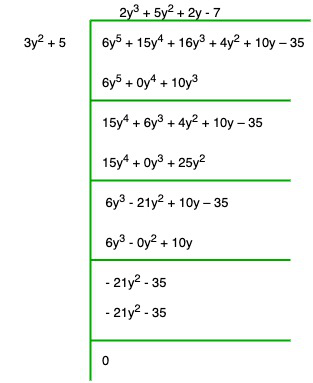Remainder = 0

Therefore, 3y2 + 5 is a factor of 6y5 + 15y4 + 16y3 + 4y2 + 10y – 35

### (v) z2 + 3 is a factor of z5– 9z

Solution:

On dividing z5 – 9z by z2 + 3, we get

-3z3 – 9z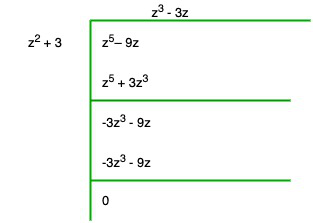Remainder = 0

Therefore, z2 + 3 is a factor of z5– 9z

### (vi) 2x2 – x + 3 is a factor of 6x5– x4 + 4x3 – 5x2 – x – 15

Solution:-

On dividing 6x5 – x4 + 4x3 – 5x2 – x – 15 by 2x2 – x + 3

-10x2 + 5x – 15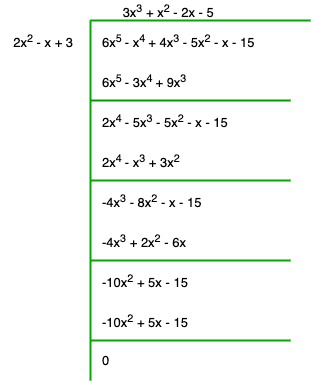Remainder = 0

Therefore, 2x2 – x + 3 is a factor of 60x5 – x4 + 4x3 – 5x2 – x – 15

### Question 24. Find the value of ‘a’, if x + 2 is a factor of 4x4 + 2x3 – 3x2 + 8x + 5a.

Solution:

Given that, x + 2 is a factor of 4x4 + 2x3 – 3x2 + 8x + 5a,

On dividing 4x4 + 2x3 – 3x2 + 8x + 5a by x + 2, we get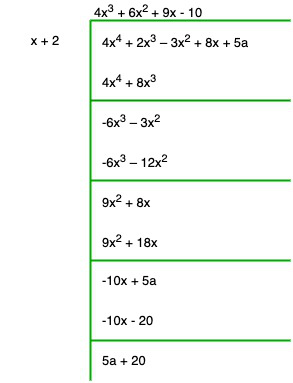Remainder = 5a + 20

5a + 20 = 0

a = -4

### Question 25. What must be added to x4 + 2x3 – 2x2 + x – 1 so that the resulting polynomial is exactly divisible by x2 + 2x – 3.

Solution:

On dividing x4 + 2x3 – 2x2 + x – 1 by x2 + 2x – 3, we get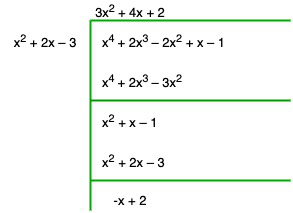Remainder = 0

The No. added to given polynomial to get remainder 0 will be :

x + 2 = 0

My Personal Notes arrow_drop_up